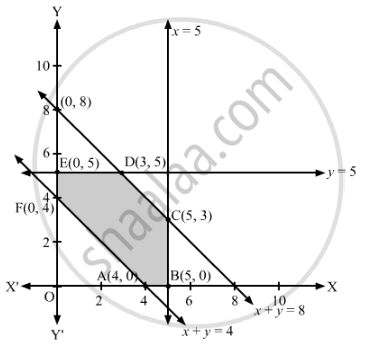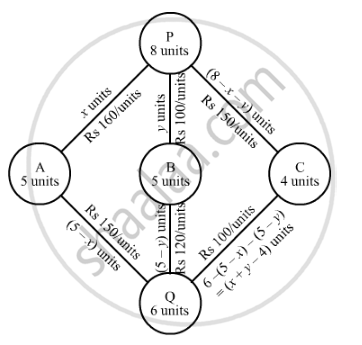# There Are Two Factories Located One at Place P and the Other at Place Q. from These Locations, a Certain Commodity is to Be Delivered to Each of the Three Depots Situated at A, B and C - Mathematics

Sum

There are two factories located one at place P and the other at place Q. From these locations, a certain commodity is to be delivered to each of the three depots situated at A, B and C. The weekly requirements of the depots are respectively 5, 5 and 4 units of the commodity while the production capacity of the factories at P and Q are respectively 8 and 6 units. The cost of transportation per unit is given below:

 From \ To Cost (in ₹) A B C P 160 100 150 Q 100 120 100

How many units should be transported from each factory to each depot in order that the transportation cost is minimum. What will be the minimum transportation cost?

#### Solution

Here, demand of the commodity (5 + 5 + 4 = 14 units) is equal the supply of the commodity (8 + 6 = 14 units). So, no commodity would be left at the two factories.

Let x units and y units of the commodity be transported from the factory P to the depots at A and B, respectively.Then, (8 − x − y) units of the commodity will be transported from the factory P to the depot C.

Now, the weekly requirement of depot A is 5 units of the commodity. Now, x units of the commodity are transported from factory P, so the remaining (5 − x) units of the commodity are transported from the factory Q to the depot A.

The weekly requirement of depot B is 5 units of the commodity. Now, y units of the commodity are transported from factory P, so the remaining (5 − y) units of the commodity are transported from the factory Q to the depot B.

Similarly, 6 − (5 − x) − (5 − y) = (x + − 4) units of the commodity will be transported from the factory Q to the depot C.

Since the number of units of commodity transported are from the factories to the depots are non-negative, therefore,

x ≥ 0, y ≥ 0, 8 − x − ≥ 0, 5 − ≥ 0, 5 − ≥ 0, x + − 4 ≥ 0

Or x ≥ 0, y ≥ 0, x + y ≤ 8, ≤ 5, ≤ 5, x + y ≥ 4

Total transportation cost = 160x + 100y + 150(8 − x − y) + 100(5 − x) + 120(5 − y) + 100(x + − 4) = 10x − 70y + 1900

Thus, the given linear programming problem is

Minimise Z = 10x − 70y + 1900

subject to the constraints

x + y ≤ 8

≤ 5

≤ 5

x + y ≥ 4

x ≥ 0, y ≥ 0

The feasible region determined by the given constraints can be diagrammatically represented as,The coordinates of the corner points of the feasible region are A(4, 0), B(5, 0), C(5, 3), D(3, 5), E(0, 5) and F(0, 4).

The value of the objective function at these points are given in the following table.

 Corner Point Z = 10x − 70y + 1900 (4, 0) 10 × 4 − 70 × 0 + 1900 = 1940 (5, 0) 10 × 5 − 70 × 0 + 1900 = 1950 (5, 3) 10 × 5 − 70 × 3 + 1900 = 1740 (3, 5) 10 × 3 − 70 × 5 + 1900 = 1580 (0, 5) 10 × 0 − 70 × 5 + 1900 = 1550 → Minimum (0, 4) 10 × 0 − 70 × 4 + 1900 = 1620

The minimum value of Z is 1550 at x = 0, y = 5.

Hence, for minimum transportation cost, factory P should supply 0, 5, 3 units of commodity to depots A, B, C respectively and factory Q should supply 5, 0, 1 units of commodity to depots A, B, C respectively.

The minimum transportation cost is ₹1,550.

Concept: Graphical Method of Solving Linear Programming Problems
Is there an error in this question or solution?

#### APPEARS IN

RD Sharma Class 12 Maths
Chapter 30 Linear programming
Exercise 30.4 | Q 49 | Page 57